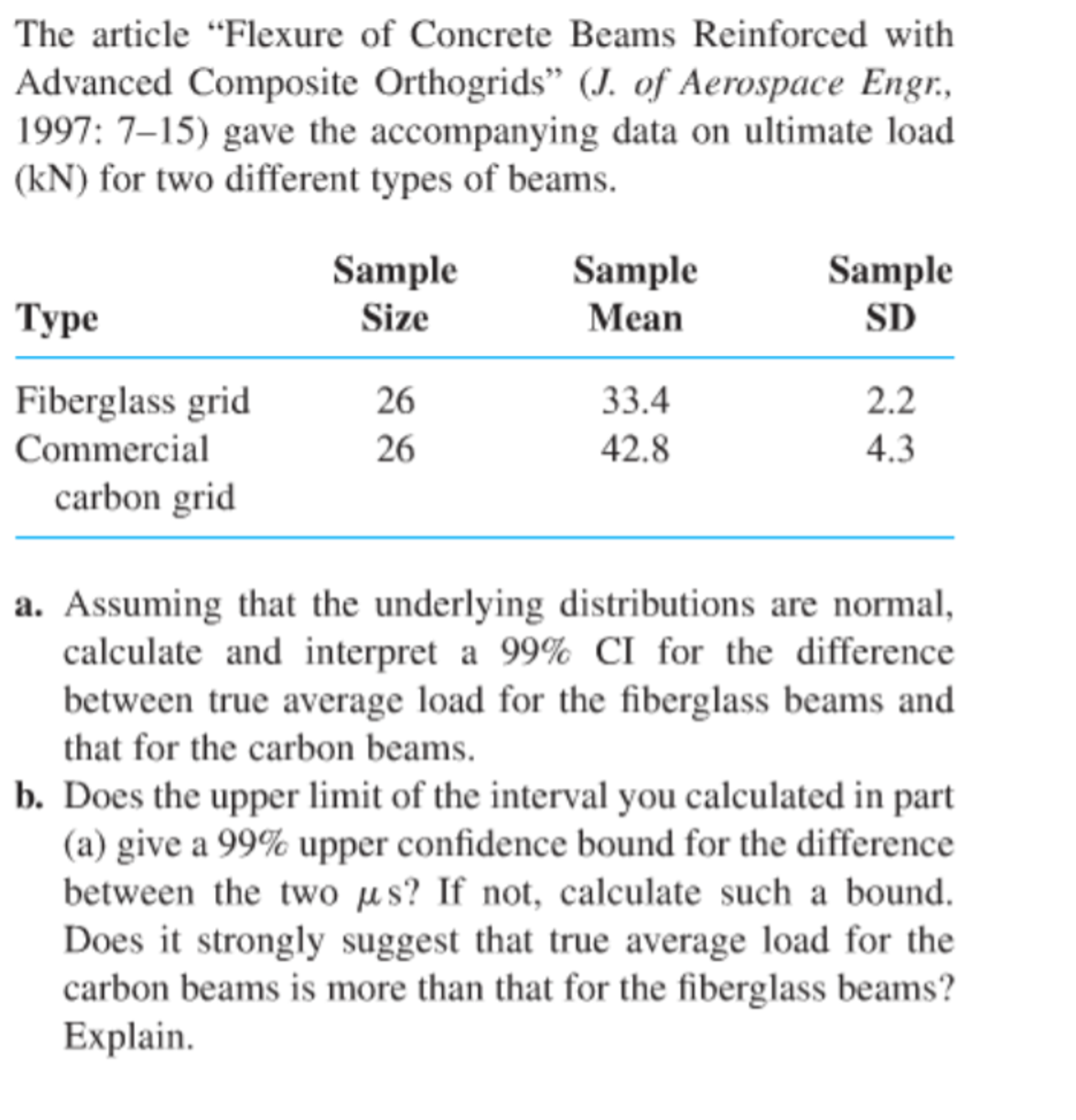# The article "Flexure of Concrete Beams Reinforced withAdvanced Composite Orthogrids" (J. of Aerospace Engr,1997: 7-15) gave the accompanying data on ultimate load(kN) for two different types of beams.SampleSampleSampleMeanТуреSDSize33.42.2Fiberglass grid262642.84.3Commercialcarbon grida. Assuming that the underlying distributions are normalcalculate and interpret a 99% CI for the differencebetween true average load for the fiberglass beams andthat for the carbon beams.b. Does the upper limit of the interval you calculated in part(a) give a 99% upper confidence bound for the differencebetween the two us? If not, calculate such a bound.Does it strongly suggest that true average load for thecarbon beams is more than that for the fiberglass beams?Explain

Questionhelp_outlineImage TranscriptioncloseThe article "Flexure of Concrete Beams Reinforced with Advanced Composite Orthogrids" (J. of Aerospace Engr, 1997: 7-15) gave the accompanying data on ultimate load (kN) for two different types of beams. Sample Sample Sample Mean Туре SD Size 33.4 2.2 Fiberglass grid 26 26 42.8 4.3 Commercial carbon grid a. Assuming that the underlying distributions are normal calculate and interpret a 99% CI for the difference between true average load for the fiberglass beams and that for the carbon beams. b. Does the upper limit of the interval you calculated in part (a) give a 99% upper confidence bound for the difference between the two us? If not, calculate such a bound. Does it strongly suggest that true average load for the carbon beams is more than that for the fiberglass beams? Explain fullscreen
check_circleExpert Solution
Step 1

Solution:

a.The 99% confidence interval for the difference between true average load for the fiberglass beams and that for the carbon beams are obtained below:

From the given information, fiberglass grid sample size is 26, sample mean is 33.4 and sample standard deviation is 2.2. The commercial carbon grid sample size is 26, sample mean is 42.8 and sample standard deviation is 4.3.

The level of significance=1-0.99=0.01.

The degrees of freedom= n1+n2-2=26+26-2=50

The critical value is,

From the EXCEL, using the formula, =T.INV.2T(0.01,50), the critical value is 2.678.

The required confidence interval is,

Step 2

Interpretation:

There is 99% confident that the difference between true average load for the fiberglass beams and that for the carbon ...

### Want to see the full answer?

See Solution

#### Want to see this answer and more?

Solutions are written by subject experts who are available 24/7. Questions are typically answered within 1 hour*

See Solution
*Response times may vary by subject and question
Tagged in

### Statistics